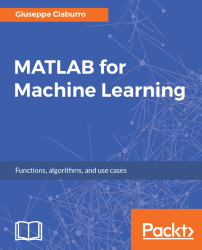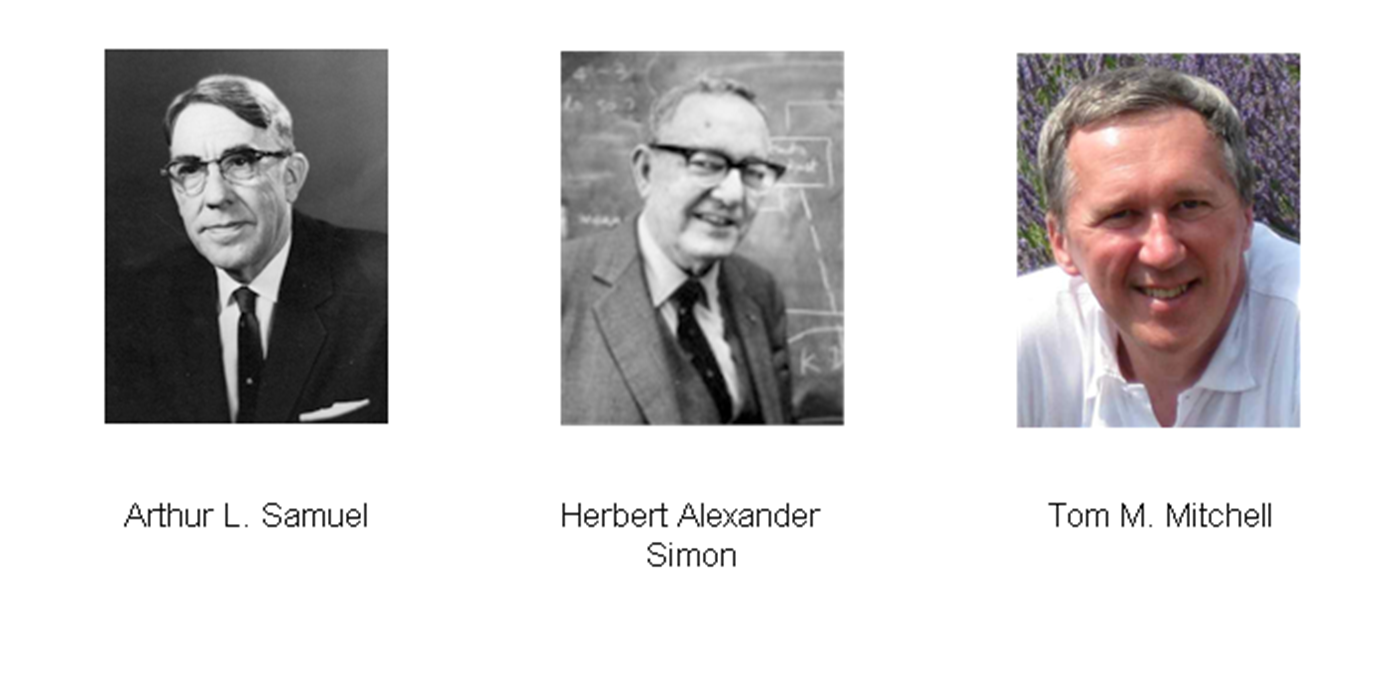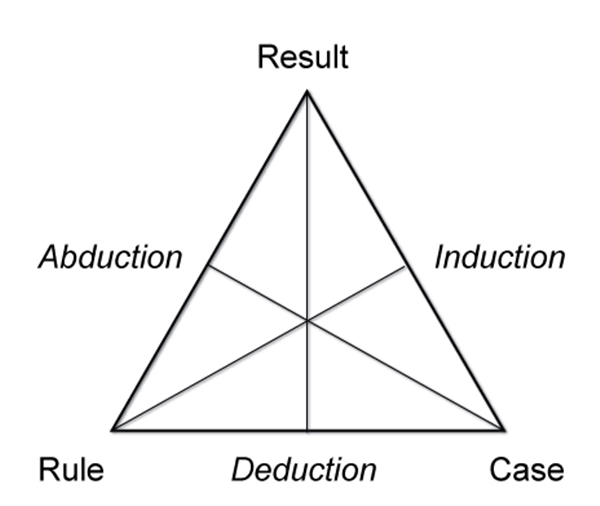•#### MATLAB for Machine Learning#### Overview of this book

MATLAB is the language of choice for many researchers and mathematics experts for machine learning. This book will help you build a foundation in machine learning using MATLAB for beginners. You’ll start by getting your system ready with t he MATLAB environment for machine learning and you’ll see how to easily interact with the Matlab workspace. We’ll then move on to data cleansing, mining and analyzing various data types in machine learning and you’ll see how to display data values on a plot. Next, you’ll get to know about the different types of regression techniques and how to apply them to your data using the MATLAB functions. You’ll understand the basic concepts of neural networks and perform data fitting, pattern recognition, and clustering analysis. Finally, you’ll explore feature selection and extraction techniques for dimensionality reduction for performance improvement. At the end of the book, you will learn to put it all together into real-world cases covering major machine learning algorithms and be comfortable in performing machine learning with MATLAB.
Title PageCreditsForewordwww.PacktPub.comCustomer FeedbackPrefaceFree Chapter
Getting Started with MATLAB Machine LearningImporting and Organizing Data in MATLABFrom Data to Knowledge DiscoveryFinding Relationships between Variables - Regression TechniquesPattern Recognition through Classification AlgorithmsIdentifying Groups of Data Using Clustering MethodsSimulation of Human Thinking - Artificial Neural NetworksImproving the Performance of the Machine Learning Model - Dimensionality ReductionMachine Learning in Practice## ABC of machine learning

Defining machine learning is not a simple matter; to do that, we can start from the definitions given by leading scientists in the field:

"Machine Learning: Field of study that gives computers the ability to learn without being explicitly programmed."                                                                                                 – Arthur L. Samuel(1959)

Otherwise, we can also provide a definition as:

"Learning denotes changes in the system that are adaptive in the sense that they enable the system to do the same task or tasks drawn from the same population more efficiently and more effectively the next time."                                                                                          – Herbert Alexander Simon (1984)

Finally, we can quote the following:

"A computer program is said to learn from experience E with respect to some class of tasks T and performance measure P, if its performance at tasks in T, as measured by P, improves with experience E".                                                                                                       – Tom M. Mitchell(1998)

In all cases, they refer to the ability to learn from experience without any outside help. Which is what we do humans in most cases. Why should it not be the same for machines?Figure 1.1: The history of machine learning

Machine learning is a multidisciplinary field created by intersection and synergy between computer science, statistics, neurobiology, and control theory. Its emergence has played a key role in several fields and has fundamentally changed the vision of software programming. If the question before was, How to program a computer? now the question becomes, How will computers program themselves?

Thus, it is clear that machine learning is a basic method that allows a computer to have its own intelligence.

As it might be expected, machine learning interconnects and coexists with the study of, and research on, human learning. Like humans, whose brain and neurons are the foundation of insight, Artificial Neural Networks (ANNs) are the basis of any decision-making activity of the computer.

From a set of data, we can find a model that describes it by the use of machine learning. For example, we can identify a correspondence between input variables and output variables for a given system. One way to do this is to postulate the existence of some kind of mechanism for the parametric generation of data, which, however, does not know the exact values of the parameters. This process typically makes reference to statistical techniques such as Induction, Deduction, and Abduction, as shown in the following figure:Figure 1.2:  Peirce’s triangle - scheme of the relationship between reasoning patterns

The extraction of general laws from a set of observed data is called induction; it is opposed to deduction, in which, starting from general laws, we want to predict the value of a set of variables. Induction is the fundamental mechanism underlying the scientific method, in which we want to derive general laws (typically described in a mathematical language) starting from the observation of phenomena.

This observation includes the measurement of a set of variables and therefore the acquisition of data that describes the observed phenomena. Then, the resulting model can be used to make predictions on additional data. The overall process in which, starting from a set of observations, we want to make predictions for new situations is called inference.

Therefore, inductive learning starts from observations arising from the surrounding environment and generalizes obtaining knowledge that will be valid for not-yet-observed cases; at least we hope so.

We can distinguish two types of inductive learning:

• Learning by example: Knowledge gained by starting from a set of positive examples that are instances of the concept to be learned and negative examples that are non-instances of the concept.
• Learning regularity: This is not a concept to learn. The goal is to find regularity (common characteristics) in the instances provided.

The following figure shows the types of inductive learning:Figure 1.3: Types of inductive learning

A question arises spontaneously: Why do machine learning systems work while traditional algorithms fail? The reasons for the failure of traditional algorithms are numerous and typically due to the following:

• Difficulty in problem formalization: For example, each of us can recognize our friends from their voice. But probably none can describe a sequence of computational steps enabling them to recognize the speaker from the recorded sound.
• High number of variables at play: When considering the problem of recognizing characters from a document, specifying all parameters that are thought to be involved can be particularly complex. In addition, the same formalization applied in the same context but on a different idiom could prove inadequate.
• Lack of theory: Imagine you have to predict exactly the performance of financial markets in the absence of specific mathematical laws.
• Need for customization: The distinction between interesting and uninteresting features depends significantly on the perception of the individual user.

Here is a flowchart showing inductive and deductive learning:Figure 1.4: Inductive and deductive learning flowchart